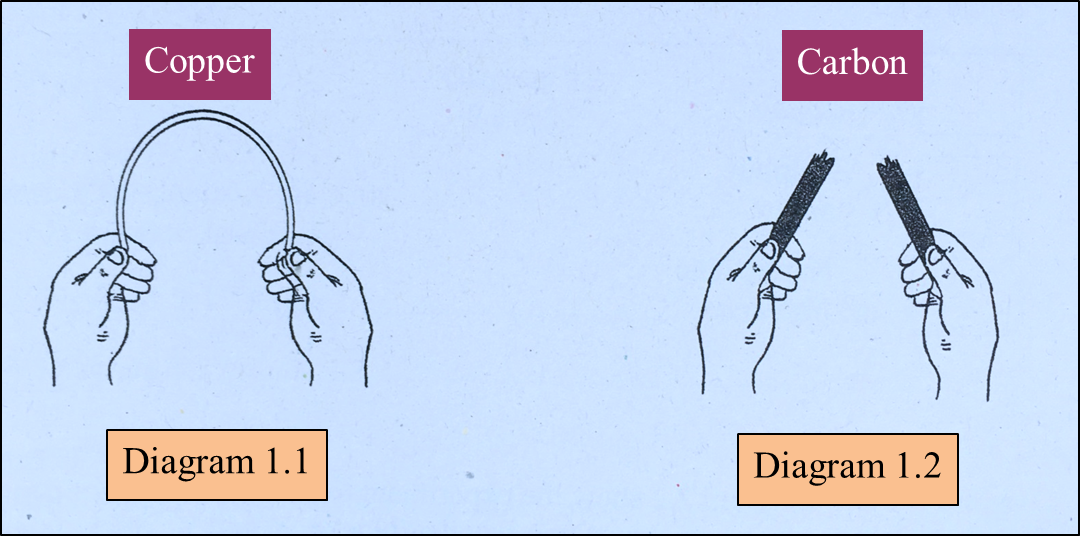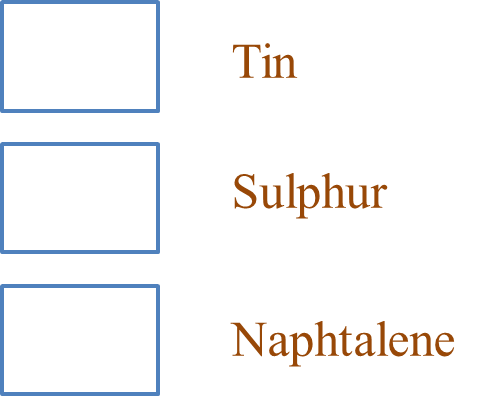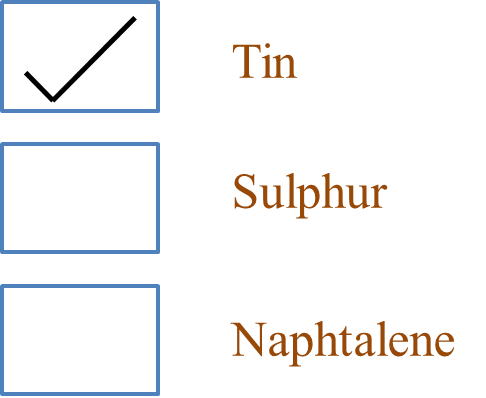# SPM Science 2018, Paper 2 (Question 1 & 2)

Question 1:
A student conducts an experiment to study the tensile strength of two types of substance. Diagram 1.1 and Diagram 1.2 show the results of the experiment.(a)
Based on Diagram 1.1 and Diagram 1.2, name the substance which has high tensile strength. [1 mark]

(b)
State one manipulated variable for this experiment. [1 mark]

(c)
State one hypothesis for this experiment. [1 mark]

(d)
Carbon is a non-metal substance. Based on this experiment, state the operational definition for non-metal. [1 mark]

(e)
Mark (\/) the substance which has the same characteristic as the substance in Diagram 1.1. [1 mark]